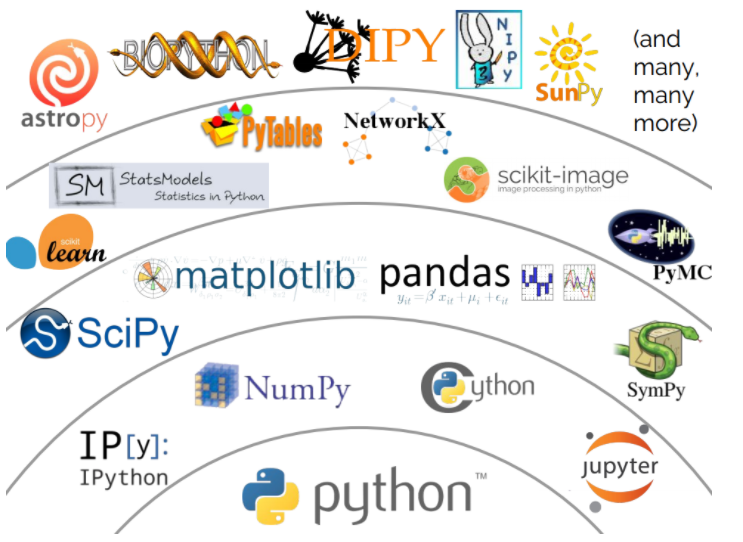Search

# An introduction to Scikit-Learn | Get Instant Help In Machine Learning

Examples require a Python distribution with scientific packages:

2. bash Anaconda2-4.2.0-Linux-x86_64.sh (or whatever installer you picked)

3. conda install scikit-learn numpy scipy matplotlib jupyter pandas

4. You are ready to go!

```# Global imports and settings

# Matplotlib
%matplotlib inline
from matplotlib import pyplot as plt
plt.rcParams["figure.max_open_warning"] = -1

# Print options
import numpy as np
np.set_printoptions(precision=3)

# Slideshow
from notebook.services.config import ConfigManager
cm = ConfigManager()
cm.update('livereveal', {'width': 1440, 'height': 768, 'scroll': True, 'theme': 'simple'})

# Silence warnings
import warnings
warnings.simplefilter(action="ignore", category=FutureWarning)
warnings.simplefilter(action="ignore", category=UserWarning)
warnings.simplefilter(action="ignore", category=RuntimeWarning)```

Outline

• Scikit-Learn and the scientific ecosystem in Python

• Supervised learning

• Transformers, pipelines and feature unions

• Beyond building classifiers

• Summary

Scikit-Learn

• Machine learning library written in Python

• Simple and efficient, for both experts and non-experts

• Classical, well-established machine learning algorithms

• Shipped with documentation and examples

Python stack for data analysis

The open source Python ecosystem provides a standalone, versatile and powerful scientific working environment, including: NumPy, SciPy, Jupyter Matplotlib, Pandas, and many others...• Scikit-Learn builds upon NumPy and SciPy and complements this scientific environment with machine learning algorithms;

• By design, Scikit-Learn is non-intrusive, easy to use and easy to combine with other libraries;

• Core algorithms are implemented in low-level languages.

Algorithms

Supervised learning:

• Linear models (Ridge, Lasso, Elastic Net, ...)

• Support Vector Machines

• Tree-based methods (Random Forests, Bagging, GBRT, ...)

• Nearest neighbors

• Neural networks

• Gaussian Processes

• Feature selection

Unsupervised learning:

• Clustering (KMeans, Ward, ...)

• Matrix decomposition (PCA, ICA, ...)

• Density estimation

• Outlier detection

Model selection and evaluation:

• Cross-validation

• Grid-search

• Lots of metrics

Supervised learningApplications

• Classifying signal from background events;

• Diagnosing disease from symptoms;

• Recognising cats in pictures;

• Identifying body parts with cameras;

• Predicting temperature for the next days

Data

• Input data = Numpy arrays or Scipy sparse matrices ;

• Algorithms are expressed using high-level operations defined on matrices or vectors (similar to MATLAB) ;

• Leverage efficient low-leverage implementations ;

• Keep code short and readable.

```# Generate data
from sklearn.datasets import make_blobs
X, y = make_blobs(n_samples=300, centers=20, random_state=123)
labels = ["b", "r"]
y = np.take(labels, (y < 10))
print(X[:5])
print(y[:5])

```

Output:

[[-2.956 -3.749]

[-7.586 2.066]

[ 0.457 8.059]

[-5.996 2.021]

[-0.979 -9.781]]

['b' 'r' 'b' 'b' 'r']

```#Print the shape
# X is a 2 dimensional array, with 300 rows and 2 columns
print(X.shape)

# y is a vector of 300 elements
print(y.shape)

```

Result:

(300, 2) (300,)

Accessing row and column

```# Rows and columns can be accessed with lists, slices or masks
print(X[[1, 2, 3]])     # rows 1, 2 and 3
print(X[:5])            # 5 first rows
print(X[200:210, 0])    # values from row 200 to row 210 at column 0
print(X[y == "b"][:5])  # 5 first rows for which y is "b"```

Output:

```[[-7.586  2.066]
[ 0.457  8.059]
[-5.996  2.021]]
[[-2.956 -3.749]
[-7.586  2.066]
[ 0.457  8.059]
[-5.996  2.021]
[-0.979 -9.781]]
[ -1.448  -6.3    -6.195  -1.99   -3.411  -7.009   5.402  -4.995  10.883
-6.661]
[[-2.956 -3.749]
[ 0.457  8.059]
[-5.996  2.021]
[-4.021 -5.173]
[ 4.01   2.581]]```

```# Plot
for label in labels:
plt.xlim(-10, 10)
plt.ylim(-10, 10)
plt.show()```

OutputA simple and unified API

All learning algorithms in scikit-learn share a uniform and limited API consisting of complementary interfaces:

• an estimator interface for building and fitting models;

• a predictor interface for making predictions;

• a transformer interface for converting data.

Goal: enforce a simple and consistent API to make it trivial to swap or plug algorithms.### Estimators

```class Estimator(object):
def fit(self, X, y=None):
"""Fits estimator to data."""
# set state of ``self``
# ...
return self```
```# Import the nearest neighbor class
from sklearn.neighbors import KNeighborsClassifier  # Change this to try
# something else

# Set hyper-parameters, for controlling algorithm
clf = KNeighborsClassifier(n_neighbors=5)

# Learn a model from training data
clf.fit(X, y)```

Result

KNeighborsClassifier(algorithm='auto', leaf_size=30, metric='minkowski', metric_params=None, n_jobs=1, n_neighbors=5, p=2, weights='uniform')

```# Estimator state is stored in instance attributes
clf._tree```

Output

<sklearn.neighbors.kd_tree.KDTree at 0x33ecde8>

Predictors

```# Make predictions
print(clf.predict(X[:5])) ```

result:

['b' 'r' 'b' 'b' 'r']

```# Compute (approximate) class probabilities
print(clf.predict_proba(X[:5]))```

result:

[[ 1. 0. ] [ 0.4 0.6] [ 1. 0. ] [ 0.6 0.4] [ 0. 1. ]]

```from tutorial import plot_surface
plot_surface(clf, X, y)```

result:```from tutorial import plot_histogram
plot_histogram(clf, X, y)```### Classifier zoo

Decision trees

Idea: greedily build a partition of the input space using cuts orthogonal to feature axes.

```from tutorial import plot_clf
from sklearn.tree import DecisionTreeClassifier
clf = DecisionTreeClassifier()
clf.fit(X, y)
plot_clf(clf, X, y)```

result:Random Forests

Idea: Build several decision trees with controlled randomness and average their decisions.

```from sklearn.ensemble import RandomForestClassifier
clf = RandomForestClassifier(n_estimators=500)
# from sklearn.ensemble import ExtraTreesClassifier
# clf = ExtraTreesClassifier(n_estimators=500)
clf.fit(X, y)
plot_clf(clf, X, y)```

result: